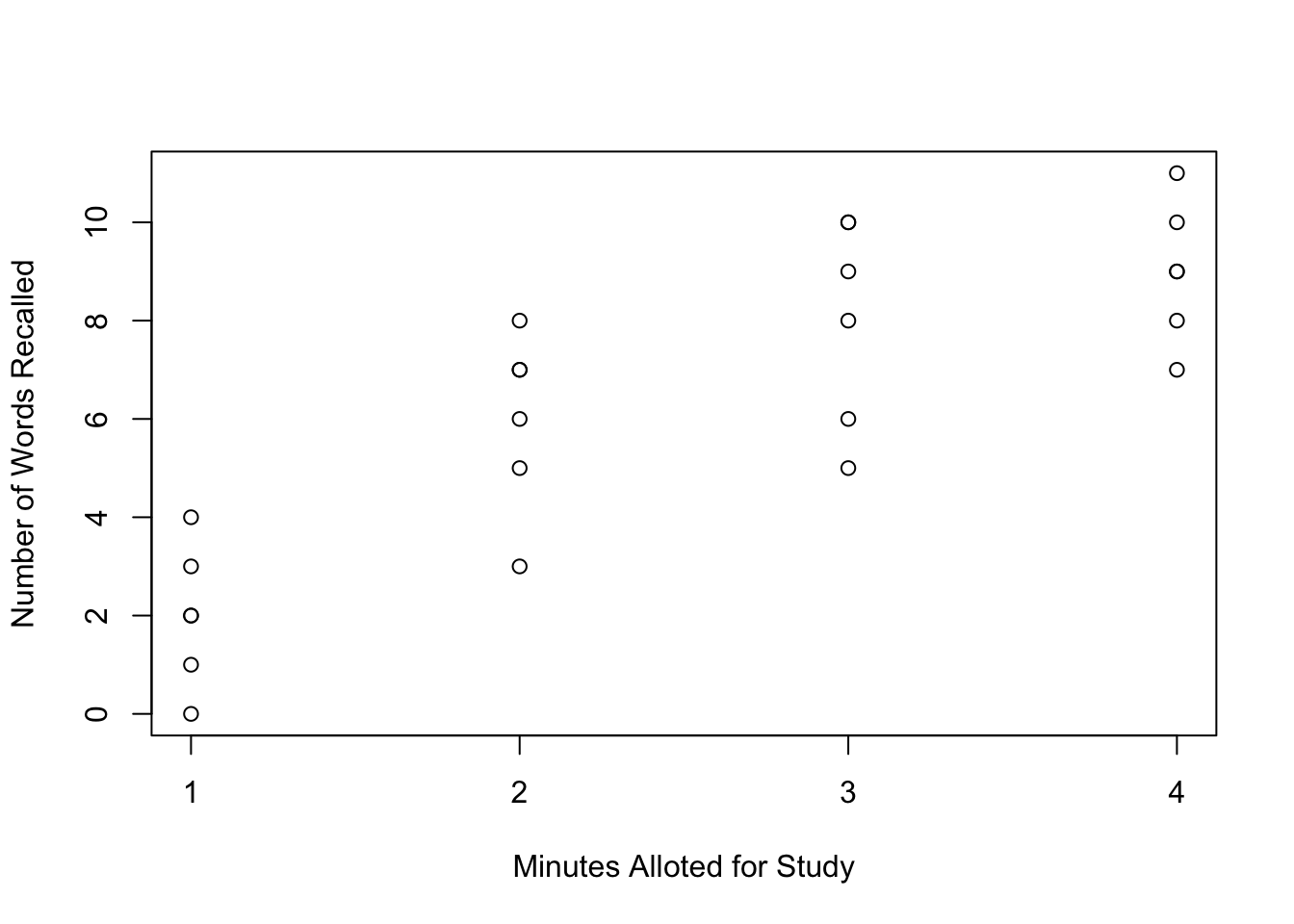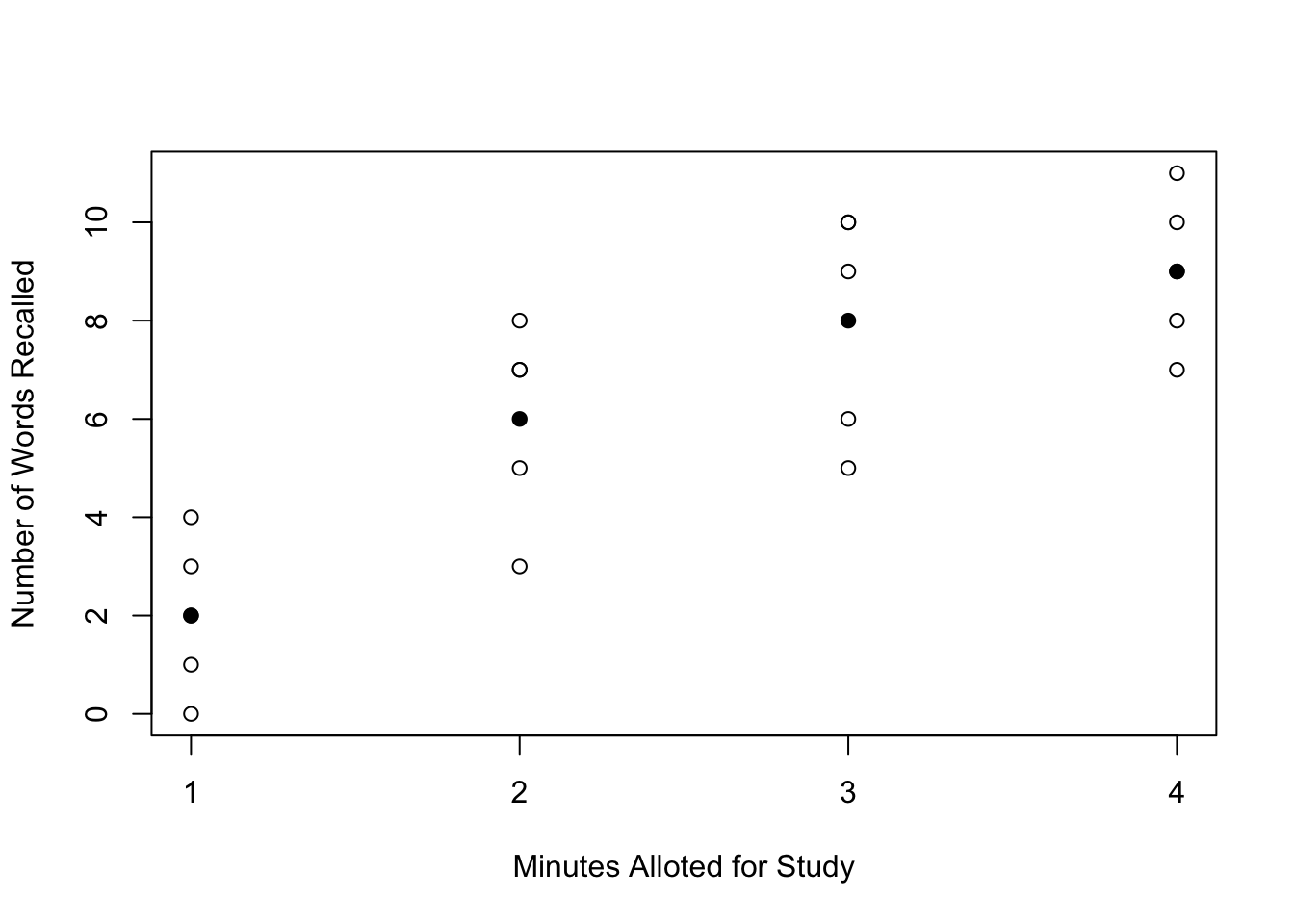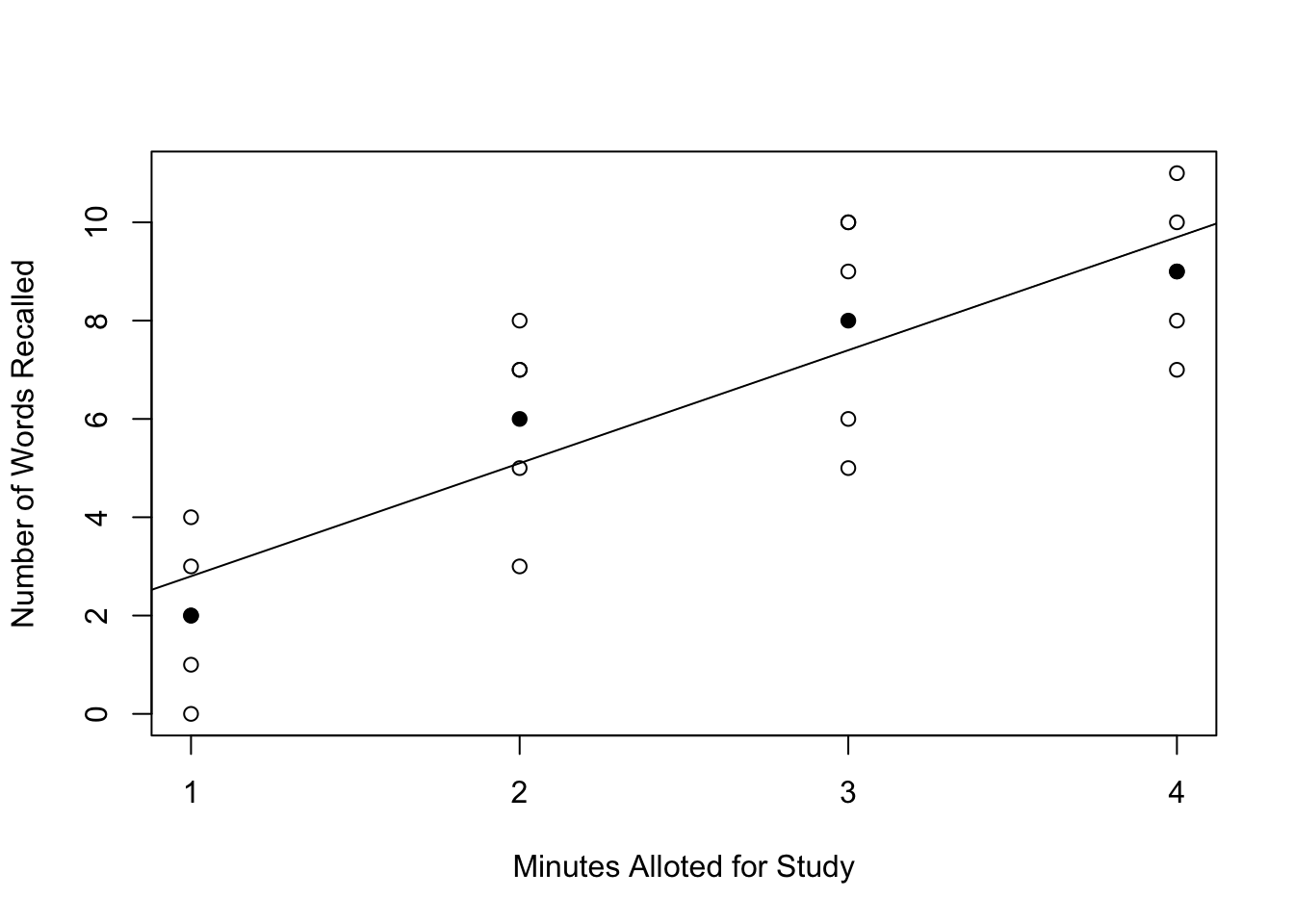# Designing Experiments and Analyzing Data: A Model Comparison Perspective (3rd edition) by Maxwell, Delaney, & Kelley

## Information about the book is available at https://designingexperiments.com

### R Code and Instructions to Accompany Chapter 6

These notes build on Chapter 1 and Chapters 3–5, where we discuss some operational aspects of R and detail the use of many functions and how to install packages. Here, we skip details that have already been discussed in the prior R Code and Instructions.

Load the AMCP package to use the data from Table 4, Chapter 5.

library(AMCP)
data(chapter_6_table_1)
chapter_6_table_1
##    Recall Minutes
## 1       2       1
## 2       3       1
## 3       1       1
## 4       2       1
## 5       0       1
## 6       4       1
## 7       6       2
## 8       8       2
## 9       5       2
## 10      3       2
## 11      7       2
## 12      7       2
## 13      6       3
## 14      8       3
## 15     10       3
## 16      5       3
## 17     10       3
## 18      9       3
## 19     11       4
## 20     10       4
## 21      7       4
## 22      9       4
## 23      8       4
## 24      9       4

Here we plot the data. In the plot() function we use xaxt="n" because we want to use tick-marks and labels only at 1, 2, 3, and 4 (by default, for this quantative data, R includes more values).

plot(Recall~Minutes, data=chapter_6_table_1, xaxt="n", xlab="Minutes Alloted for Study", ylab="Number of Words Recalled")
axis(1, at=1:4, labels=1:4)Standard ANOVA appraoch (ignoring the quantitative nature of Minutes)

ANOVA.Object <- aov(Recall~as.factor(Minutes), data=chapter_6_table_1)
ANOVA_Result <- anova(ANOVA.Object)
ANOVA_Result
## Analysis of Variance Table
##
## Response: Recall
##                    Df Sum Sq Mean Sq F value    Pr(>F)
## as.factor(Minutes)  3  172.5    57.5  19.828 3.306e-06 ***
## Residuals          20   58.0     2.9
## ---
## Signif. codes:  0 '***' 0.001 '**' 0.01 '*' 0.05 '.' 0.1 ' ' 1

Regression appraoch

Regression.Object <- lm(Recall~Minutes, data=chapter_6_table_1)
Regression_Result <- summary(Regression.Object)
Regression_Result
##
## Call:
## lm(formula = Recall ~ Minutes, data = chapter_6_table_1)
##
## Residuals:
##    Min     1Q Median     3Q    Max
## -2.800 -1.475  0.050  1.375  2.900
##
## Coefficients:
##             Estimate Std. Error t value Pr(>|t|)
## (Intercept)   0.5000     0.9033   0.554    0.585
## Minutes       2.3000     0.3298   6.973 5.33e-07 ***
## ---
## Signif. codes:  0 '***' 0.001 '**' 0.01 '*' 0.05 '.' 0.1 ' ' 1
##
## Residual standard error: 1.807 on 22 degrees of freedom
## Multiple R-squared:  0.6885, Adjusted R-squared:  0.6743
## F-statistic: 48.63 on 1 and 22 DF,  p-value: 5.333e-07

Form summary values of interest

library(dplyr)
##
## Attaching package: 'dplyr'
## The following objects are masked from 'package:stats':
##
##     filter, lag
## The following objects are masked from 'package:base':
##
##     intersect, setdiff, setequal, union
Summary.Recall <- chapter_6_table_1 %>%
group_by(Minutes) %>%
summarize(
mean.Recall=mean(Recall),
sd.Recall=sd(Recall),
n.Recall=n()
)
Summary.Recall
## # A tibble: 4 × 4
##   Minutes mean.Recall sd.Recall n.Recall
##     <int>       <dbl>     <dbl>    <int>
## 1       1           2  1.414214        6
## 2       2           6  1.788854        6
## 3       3           8  2.097618        6
## 4       4           9  1.414214        6
# Define the c-weights for the linear contrast
Summary.Recall$c.weight <- Summary.Recall$Minutes - mean(chapter_6_table_1$Minutes) Summary.Recall ## # A tibble: 4 × 5 ## Minutes mean.Recall sd.Recall n.Recall c.weight ## <int> <dbl> <dbl> <int> <dbl> ## 1 1 2 1.414214 6 -1.5 ## 2 2 6 1.788854 6 -0.5 ## 3 3 8 2.097618 6 0.5 ## 4 4 9 1.414214 6 1.5 Summary.Minutes <- c(mean=mean(chapter_6_table_1$Minutes), sd=sd(chapter_6_table_1$Minutes)) Summary.Minutes ## mean sd ## 2.50000 1.14208 Form linear contrast. C.Linear <- Summary.Recall$c.weight
MS.W <- ANOVA_Result$"Mean Sq" Psi.Linear <- sum(Summary.Recall$mean.Recall*C.Linear)
Psi.Linear
##  11.5
# Equation 7
b1 <- Psi.Linear/sum(C.Linear^2)
b1
##  2.3

Plot with mean noted (solid circle) at each minute studied.

plot(Recall~Minutes, data=chapter_6_table_1, xaxt="n", xlab="Minutes Alloted for Study", ylab="Number of Words Recalled")
axis(1, at=1:4, labels=1:4)
points(Summary.Recall$mean.Recall ~ Summary.Recall$Minutes, pch=16)Estimate the intercept

b0 <- mean(chapter_6_table_1$Recall) - b1*mean(chapter_6_table_1$Minutes)
b0
##  0.5

Plot with group mean and linear trend included.

plot(Recall~Minutes, data=chapter_6_table_1, xaxt="n", xlab="Minutes Alloted for Study", ylab="Number of Words Recalled")
axis(1, at=1:4, labels=1:4)
points(Summary.Recall$mean.Recall ~ Summary.Recall$Minutes, pch=16)
abline(a=b0, b=b1)Form sum of squares of the linear contrast.

#Repeated
C.Linear <- Summary.Recall$c.weight MS.W <- ANOVA_Result$"Mean Sq"

SS.Linear <- Psi.Linear^2/(sum(C.Linear^2/Summary.Recall$n)) ## Warning: Unknown column 'n' SS.Linear ##  Inf F.Linear <- SS.Linear/MS.W F.Linear ##  Inf An alternative appraoch is to use the ci.c() function from MBESS (as was used in Chapter 4) to obtain confidence intervals. In the first use below we use the c-weights directly, whereas in the second appraoch we scale the c-weights by dividing by the sum of the squared c-weights. library(MBESS) # Using raw c-weights for the linear effect. ci.c(means=Summary.Recall$mean.Recall, c.weights=Summary.Recall$c.weight, n=Summary.Recall$n.Recall, N=sum(Summary.Recall$n.Recall), s.anova=sqrt(MS.W)) ##$Lower.Conf.Limit.Contrast
##  8.257238
##
## $Contrast ##  11.5 ## ##$Upper.Conf.Limit.Contrast
##  14.74276
# Using the scaled c-weights for the linear effect.
ci.c(means=Summary.Recall$mean.Recall, c.weights=Summary.Recall$c.weight/sum(Summary.Recall$c.weight^2), n=Summary.Recall$n.Recall, N=sum(Summary.Recall$n.Recall), s.anova=sqrt(MS.W)) ##$Lower.Conf.Limit.Contrast
##  1.651448
##
## $Contrast ##  2.3 ## ##$Upper.Conf.Limit.Contrast
##  2.948552

Now we consider a more program based appraoch to trend analysis. In R, the contr.poly() function provides contrast matricies for such purposes as trend analysis (with some additional programing, deomonstrated below). For j trend levels, j is used within the contr.poly() function as contr.poly(j), where a matrix of orthogonal polynomial coefficients in a j by j-1 matrix will be returned. The use of contr.poly() returns numbers that are different than the book (which uses c-weights that seem immediately interpretable), but which nevertheless are simply rescalled. I

contr.poly(4)
##              .L   .Q         .C
## [1,] -0.6708204  0.5 -0.2236068
## [2,] -0.2236068 -0.5  0.6708204
## [3,]  0.2236068 -0.5 -0.6708204
## [4,]  0.6708204  0.5  0.2236068

In order to perform a trend analysis in R, we first define a new varaible, Minutes_factor which is simply Minutes as a factor (which we did before directly in the aov() function). Then, we create a new variable that defines Minutes_factor as a four-levels polynomial contrast. Examination of the data does not reveal any changes from what would be expected (see below) but when we examine the structure of the data the polynomial contrasts become clear.

chapter_6_table_1$Minutes_factor <- as.factor(chapter_6_table_1$Minutes)
contrasts(chapter_6_table_1$Minutes_factor) <- contr.poly(4) chapter_6_table_1 ## Recall Minutes Minutes_factor ## 1 2 1 1 ## 2 3 1 1 ## 3 1 1 1 ## 4 2 1 1 ## 5 0 1 1 ## 6 4 1 1 ## 7 6 2 2 ## 8 8 2 2 ## 9 5 2 2 ## 10 3 2 2 ## 11 7 2 2 ## 12 7 2 2 ## 13 6 3 3 ## 14 8 3 3 ## 15 10 3 3 ## 16 5 3 3 ## 17 10 3 3 ## 18 9 3 3 ## 19 11 4 4 ## 20 10 4 4 ## 21 7 4 4 ## 22 9 4 4 ## 23 8 4 4 ## 24 9 4 4 str(chapter_6_table_1) ## 'data.frame': 24 obs. of 3 variables: ##$ Recall        : int  2 3 1 2 0 4 6 8 5 3 ...
##  $Minutes : int 1 1 1 1 1 1 2 2 2 2 ... ##$ Minutes_factor: Factor w/ 4 levels "1","2","3","4": 1 1 1 1 1 1 2 2 2 2 ...
##   ..- attr(*, "contrasts")= num [1:4, 1:3] -0.671 -0.224 0.224 0.671 0.5 ...
##   .. ..- attr(*, "dimnames")=List of 2
##   .. .. ..$: chr "1" "2" "3" "4" ## .. .. ..$ : chr  ".L" ".Q" ".C"

We then use the aov() function as before to perform an ANOVA. Notice that use of summary() on the aov() object appears no different than before.

ANOVA.Object.Contr <- aov(Recall~Minutes_factor, data=chapter_6_table_1)
summary(ANOVA.Object.Contr)
##                Df Sum Sq Mean Sq F value   Pr(>F)
## Minutes_factor  3  172.5    57.5   19.83 3.31e-06 ***
## Residuals      20   58.0     2.9
## ---
## Signif. codes:  0 '***' 0.001 '**' 0.01 '*' 0.05 '.' 0.1 ' ' 1

Importantly, we now need to use summary() in a slightly different way, where we specify that the Minutes_factor should be split into three parts (one per degree of freedom). Now, the sum of squares for the Minutes factor (i.e., 172.5) is partitioned into the three constrasts (linear [SS=158.7], quadratic [SS=13.5], cubic [SS=.3]) because there are four levels. Notice that the sum of the two sums of squres for C2 (quadratic) and C3 (cubic) (i.e., 13.5 + .3 = 13.8) and, with two degrees of freedom, collectively test the nonlinear trends.

r summary(ANOVA.Object.Contr, split=list(Minutes_factor=1:3))

## Df Sum Sq Mean Sq F value Pr(>F) ## Minutes_factor 3 172.5 57.5 19.828 3.31e-06 *** ## Minutes_factor: C1 1 158.7 158.7 54.724 3.82e-07 *** ## Minutes_factor: C2 1 13.5 13.5 4.655 0.0433 * ## Minutes_factor: C3 1 0.3 0.3 0.103 0.7511 ## Residuals 20 58.0 2.9 ## --- ## Signif. codes: 0 '***' 0.001 '**' 0.01 '*' 0.05 '.' 0.1 ' ' 1

# Comparison to a regression appraoch.

A regression appraoch for the linear term (results repeated) along shows the confidence intervals for the coefficients can be given as follows. Note that this appraoch is for the linear term along and, for example, if one fitted a quadratic or cubic regression model the slope term will differ (in each model, generally). Note that the intervals here are different because they are from a different type of model (here, only linearity is considered but in the ANOVA implicetely the quadratic and cubic terms are also included (and thus the error term decreased accordingly))

# Recall the regression appraoch for a linear only model, and the results:
summary(Regression.Object)
##
## Call:
## lm(formula = Recall ~ Minutes, data = chapter_6_table_1)
##
## Residuals:
##    Min     1Q Median     3Q    Max
## -2.800 -1.475  0.050  1.375  2.900
##
## Coefficients:
##             Estimate Std. Error t value Pr(>|t|)
## (Intercept)   0.5000     0.9033   0.554    0.585
## Minutes       2.3000     0.3298   6.973 5.33e-07 ***
## ---
## Signif. codes:  0 '***' 0.001 '**' 0.01 '*' 0.05 '.' 0.1 ' ' 1
##
## Residual standard error: 1.807 on 22 degrees of freedom
## Multiple R-squared:  0.6885, Adjusted R-squared:  0.6743
## F-statistic: 48.63 on 1 and 22 DF,  p-value: 5.333e-07
# Confidence intervals for the coefficients can be obtained using the confint() function.
confint(Regression.Object)
##                 2.5 %   97.5 %
## (Intercept) -1.373282 2.373282
## Minutes      1.615974 2.984026

If we fit a quadratic regression model (note that the I() function can be used to perform calculations within a formula).

Regression.Object2 <- lm(Recall~Minutes + I(Minutes^2), data=chapter_6_table_1)
summary(Regression.Object2)
##
## Call:
## lm(formula = Recall ~ Minutes + I(Minutes^2), data = chapter_6_table_1)
##
## Residuals:
##    Min     1Q Median     3Q    Max
## -3.150 -0.975  0.050  1.150  2.150
##
## Coefficients:
##              Estimate Std. Error t value Pr(>|t|)
## (Intercept)   -3.2500     1.8937  -1.716  0.10083
## Minutes        6.0500     1.7275   3.502  0.00212 **
## I(Minutes^2)  -0.7500     0.3401  -2.205  0.03873 *
## ---
## Signif. codes:  0 '***' 0.001 '**' 0.01 '*' 0.05 '.' 0.1 ' ' 1
##
## Residual standard error: 1.666 on 21 degrees of freedom
## Multiple R-squared:  0.7471, Adjusted R-squared:  0.723
## F-statistic: 31.01 on 2 and 21 DF,  p-value: 5.389e-07
confint(Regression.Object2)
##                  2.5 %      97.5 %
## (Intercept)  -7.188062  0.68806206
## Minutes       2.457378  9.64262231
## I(Minutes^2) -1.457297 -0.04270317

If we fit a cubic regression model as well.

Regression.Object3 <- lm(Recall~Minutes + I(Minutes^2) + I(Minutes^3), data=chapter_6_table_1)
summary(Regression.Object3)
##
## Call:
## lm(formula = Recall ~ Minutes + I(Minutes^2) + I(Minutes^3),
##     data = chapter_6_table_1)
##
## Residuals:
##    Min     1Q Median     3Q    Max
##     -3     -1      0      1      2
##
## Coefficients:
##              Estimate Std. Error t value Pr(>|t|)
## (Intercept)   -5.0000     5.7749  -0.866    0.397
## Minutes        8.8333     8.8320   1.000    0.329
## I(Minutes^2)  -2.0000     3.9019  -0.513    0.614
## I(Minutes^3)   0.1667     0.5182   0.322    0.751
##
## Residual standard error: 1.703 on 20 degrees of freedom
## Multiple R-squared:  0.7484, Adjusted R-squared:  0.7106
## F-statistic: 19.83 on 3 and 20 DF,  p-value: 3.306e-06
confint(Regression.Object3)
##                   2.5 %    97.5 %
## (Intercept)  -17.046326  7.046326
## Minutes       -9.589944 27.256610
## I(Minutes^2) -10.139268  6.139268
## I(Minutes^3)  -0.914254  1.247587

# Numerical example

library(dplyr)

Summary.Results <- chapter_6_table_1 %>%
group_by(Minutes) %>%
summarize(
mean=mean(Recall),
sd=sd(Recall),
n=n())

c.Linear <- Summary.Results$Minutes - mean(chapter_6_table_1$Minutes)
c.Linear_Scaled <- c.Linear/sum(c.Linear^2)

Ybar <- Summary.Results$mean n <- Summary.Results$n

Psi.Linear <- sum(c.Linear_Scaled*Ybar)
Psi.Linear
##  2.3
SS.Linear <- Psi.Linear^2/sum(c.Linear_Scaled^2/n)
SS.Linear
##  158.7
F.value <- SS.Linear/MS.W
F.value
##  54.72414